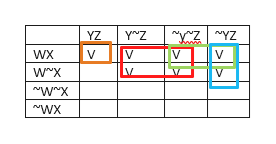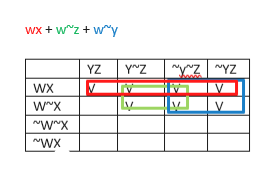Which of the following is NOT logically equivalent to the rest:wx~y + w~z + wxyz + w~yzw(x + ~y + ~z)w + x + ~y + ~zwx + w~y + w~zPlease explain all steps

Question
1. Which of the following is NOT logically equivalent to the rest:
1. wx~y + w~z + wxyz + w~yz
2. w(x + ~y + ~z)
3. w + x + ~y + ~z
4. wx + w~y + w~z

Please explain all steps

Step 1

Using a Karnaugh Map to find the minimal sum-of-products for the expressions we get the following (cells covered by the terms in the expression are marked by a ‘V’:

For expression (1) the 4 terms are shown by different colours:help_outlineImage TranscriptioncloseZYZ V YZ Y Z ww V WX V WX WX fullscreen
Step 2

So finding the minimal cover as shown by the rectangles below.

So a ...help_outlineImage Transcriptionclosewx w zwy ZYZ YZ Y Z WX wx wx WX fullscreen

Want to see the full answer?

See Solution

Want to see this answer and more?

Our solutions are written by experts, many with advanced degrees, and available 24/7

See Solution
Tagged in

Discrete Mathematics